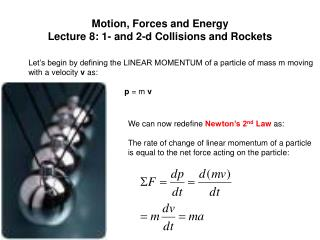DownloadDownload PresentationMotion, Forces and Energy Lecture 8: 1- and 2-d Collisions and Rockets

# Motion, Forces and Energy Lecture 8: 1- and 2-d Collisions and Rockets

Télécharger la présentation## Motion, Forces and Energy Lecture 8: 1- and 2-d Collisions and Rockets

- - - - - - - - - - - - - - - - - - - - - - - - - - - E N D - - - - - - - - - - - - - - - - - - - - - - - - - - -
##### Presentation Transcript

1. Motion, Forces and EnergyLecture 8: 1- and 2-d Collisions and Rockets Let’s begin by defining the LINEAR MOMENTUM of a particle of mass m moving with a velocity v as: p = m v We can now redefine Newton’s 2nd Law as: The rate of change of linear momentum of a particle is equal to the net force acting on the particle:

2. Conservation of Momentum For A Two-Particle System Law of Conservation of Momentum (LCM): p1i + p2i = p1f + p2f An astronaut in space wants to move. She throws her jacket in the opposite direction to that in which she wishes to move: v1f m1 v2f m2

3. v1i v2i Before collision v1f v2f After collision Elastic and Inelastic Collisions in One Dimension Momentum is conserved in any collision in which external forces are negligible. However, kinetic energy may (elastic) or may not (inelastic) be conserved. Using Law of Conservation of Momentum andConservation of Kinetic Energy (elastic collision), we can show that: Case 1: If m1=m2, v1f=v2i & v2f=v1i (exchange of speeds: pool/snooker). Case 2: If v2i=0 (initially) then (a) if m1>>m2, v1f~v1i and v2f~2v1i & (b) if m2>>m1, v1f~-v1i and v2f~v2i=0

4. v1i v2i Before collision vf After collision Perfectly Inelastic Collisions These are collisions in which the colliding objects actually stick together (merge) during the collision; thus after the collision, there is only one mass (m1+m2) involved.

5. vf v1i m1+m2 m1 m2 Dh The Ballistic Pendulum This is a device used to measure the speed of a fast-moving object such as a bullet. Since KEafter is converted into PE, we can show that:

6. An Aside: Rocket Propulsion Rocket moves upwards due to LCM Since exhaust gases are expelled downwards. vR vex We can’t write the above since the mass of the rocket+fuel is continually decreasing! So we consider a time period Dt at the beginning of which we say that the mass of the “rocket plus fuel” is MR+Dm where Dm is the mass of the emitted fuel gases in the period Dt. Also at the start of Dt, the velocity of the rocket is VR.

7. v1fsinq v1f v1fcosq Before collision v1i q f m1 m2 After collision v2fcosq v2f -v2fsinq Two-dimensional Collisions The physics behind collisions in two dimensions is the same as we have seen already, except that here we must resolve into x and y components of momentum:

8. v1fsinq v1f v1fcosq Before collision v1i q f m1 m2 v2fcosq After collision v2f -v2fsinq Resolving in x and y directions Example: A pool ball moving at 3.4 ms-1 strikes a stationary ball such that the first ball continues at a speed of 2.5 ms-1 at an angle of 30o to its original direction. Ignoring friction, rotational motion and assuming the collision is perfectly elastic, find the velocity of the struck ball and its direction. m1=m2